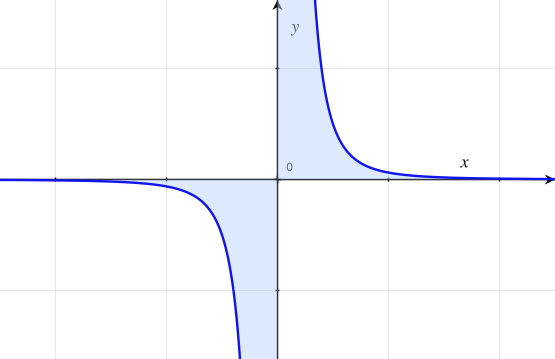# Trigonometrical Integrals$\large \displaystyle \int_{0}^{\pi} \dfrac{1}{1+ \tan x} dx$

Evaluate the Cauchy principal value of the above integral. If your answer comes as $\dfrac{\pi}{A}$, then find the value of $A$.

×